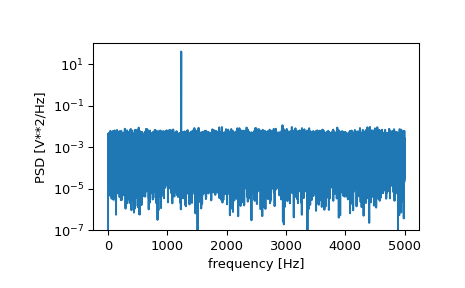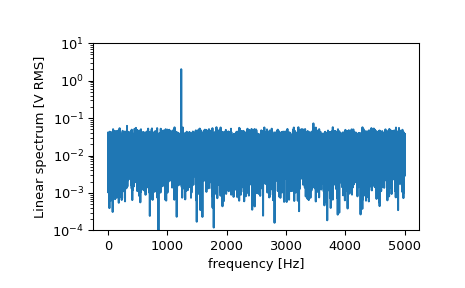# scipy.signal.periodogram¶

scipy.signal.periodogram(x, fs=1.0, window='boxcar', nfft=None, detrend='constant', return_onesided=True, scaling='density', axis=- 1)[source]

Estimate power spectral density using a periodogram.

Parameters
xarray_like

Time series of measurement values

fsfloat, optional

Sampling frequency of the x time series. Defaults to 1.0.

windowstr or tuple or array_like, optional

Desired window to use. If window is a string or tuple, it is passed to `get_window` to generate the window values, which are DFT-even by default. See `get_window` for a list of windows and required parameters. If window is array_like it will be used directly as the window and its length must be nperseg. Defaults to ‘boxcar’.

nfftint, optional

Length of the FFT used. If None the length of x will be used.

detrendstr or function or False, optional

Specifies how to detrend each segment. If `detrend` is a string, it is passed as the type argument to the `detrend` function. If it is a function, it takes a segment and returns a detrended segment. If `detrend` is False, no detrending is done. Defaults to ‘constant’.

return_onesidedbool, optional

If True, return a one-sided spectrum for real data. If False return a two-sided spectrum. Defaults to True, but for complex data, a two-sided spectrum is always returned.

scaling{ ‘density’, ‘spectrum’ }, optional

Selects between computing the power spectral density (‘density’) where Pxx has units of V**2/Hz and computing the power spectrum (‘spectrum’) where Pxx has units of V**2, if x is measured in V and fs is measured in Hz. Defaults to ‘density’

axisint, optional

Axis along which the periodogram is computed; the default is over the last axis (i.e. `axis=-1`).

Returns
fndarray

Array of sample frequencies.

Pxxndarray

Power spectral density or power spectrum of x.

`welch`

Estimate power spectral density using Welch’s method

`lombscargle`

Lomb-Scargle periodogram for unevenly sampled data

Notes

New in version 0.12.0.

Examples

```>>> from scipy import signal
>>> import matplotlib.pyplot as plt
>>> rng = np.random.default_rng()
```

Generate a test signal, a 2 Vrms sine wave at 1234 Hz, corrupted by 0.001 V**2/Hz of white noise sampled at 10 kHz.

```>>> fs = 10e3
>>> N = 1e5
>>> amp = 2*np.sqrt(2)
>>> freq = 1234.0
>>> noise_power = 0.001 * fs / 2
>>> time = np.arange(N) / fs
>>> x = amp*np.sin(2*np.pi*freq*time)
>>> x += rng.normal(scale=np.sqrt(noise_power), size=time.shape)
```

Compute and plot the power spectral density.

```>>> f, Pxx_den = signal.periodogram(x, fs)
>>> plt.semilogy(f, Pxx_den)
>>> plt.ylim([1e-7, 1e2])
>>> plt.xlabel('frequency [Hz]')
>>> plt.ylabel('PSD [V**2/Hz]')
>>> plt.show()
```If we average the last half of the spectral density, to exclude the peak, we can recover the noise power on the signal.

```>>> np.mean(Pxx_den[25000:])
0.000985320699252543
```

Now compute and plot the power spectrum.

```>>> f, Pxx_spec = signal.periodogram(x, fs, 'flattop', scaling='spectrum')
>>> plt.figure()
>>> plt.semilogy(f, np.sqrt(Pxx_spec))
>>> plt.ylim([1e-4, 1e1])
>>> plt.xlabel('frequency [Hz]')
>>> plt.ylabel('Linear spectrum [V RMS]')
>>> plt.show()
```The peak height in the power spectrum is an estimate of the RMS amplitude.

```>>> np.sqrt(Pxx_spec.max())
2.0077340678640727
```